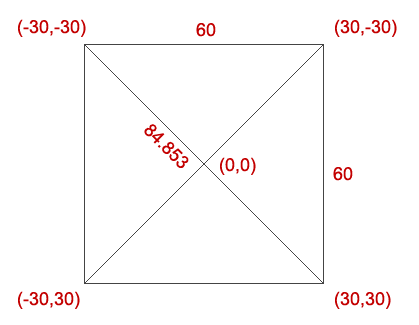# Understanding AS3 localToGlobal method

One AS3 DisplayObject method I don’t see that much used since AS2 became obsolete is `localToGlobal`.

Now I’ll show you how useful can be this method when it’s time to save coding time.

First, the definition:

localToGlobal(point:Point):Point
Converts the point object from the display object’s (local) coordinates to the Stage (global) coordinates.

Let’s see what does it mean.

We have a square, whose width (and height) is 60 pixels.Assuming its center is at (0,0), we can easily determine the coordinates of the four vertexs since if the width is 60, the half-width will be 30.

The diagonal, useful during the script you’re about to see, is dermined by:

`square_root(60*60+60*60)` or `square_root(2)*60`

Now imagine to rotate clockwise the square by an arbitrary angle, and tell me the position of the upper left vertex.

You can do it with trigonometry this way:

`x = -42.426*cos(45-angle)` = -30 if angle is zero. (42.426 is half the diagonal)
`y = -42.426*sin(45-angle)` = -30 if angle is zero

It’s not impossible, but it can be done in an easier way using `localToGlobal`.

Basically we know the upper left vertex is always at `(-30,-30`) in a world relative just the square itself, no matter of its position in the stage.

localToGlobal will handle the position in the stage according to the absolute `(-30,-30)` coordinate.

Here it is the script:

```package {
import flash.display.Sprite;
import flash.geom.Point;
public class square_mc extends Sprite {
public var diagonal:Number=42.42640687119285;
public var point:Point;
public var pos_x,pos_y:Number;
public function square_mc():void {

}
public function random_pos():void {
x=Math.random()*400+50;
y=Math.random()*300+50;
rotation=Math.random()*360;
point=new Point(-30,-30);
point=localToGlobal(point);
}
}
}```

Line 5: defining the half diagonal of a square whose width is `30`

Line 6: degrees to radians conversion number

Line 7: defining a `Point` variable. The `Point` object represents a location in a two-dimensional coordinate system, where `x` represents the horizontal axis and `y` represents the vertical axis.

Lines 9-11: intentionally left blank for a possible more complex example in the future

Lines 13-15: randomly placing and rotating the square on the stage

Lines 16-17: retrieving upper left vertex coordinates using `localToGlobal`

Lines 18-19: retrieving upper left vertex coordinates using trigonometry

Here it is the result (included in a main class that just handles input and output)

Click the mouse on the stage to randomly move the square and retrieve the upper left vertex (marked in red) using both ways.

Which one do you prefer?

214 GAME PROTOTYPES EXPLAINED WITH SOURCE CODE
// 1+2=3
// 10000000
// 2 Cars
// 2048
// Avoider
// Ballz
// Block it
// Blockage
// Bloons
// Boids
// Bombuzal
// Breakout
// Bricks
// Columns
// CubesOut
// Dots
// DROP'd
// Dudeski
// Eskiv
// Filler
// Fling
// Globe
// HookPod
// Hundreds
// InkTd
// Iromeku
// Lumines
// Magick
// MagOrMin
// Maze
// Memdot
// Nano War
// Nodes
// o:anquan
// Ononmin
// Pacco
// Phyballs
// Platform
// Poker
// Pool
// Poux
// Pudi
// qomp
// Racing
// Renju
// SameGame
// Security
// Sling
// Slingy
// Sokoban
// Splitter
// Sproing
// Stack
// Stringy
// Sudoku
// Tetris
// Threes
// Toony
// Turn
// TwinSpin
// vvvvvv
// Wordle
// Worms
// Yanga
// Zhed
// zNumbers